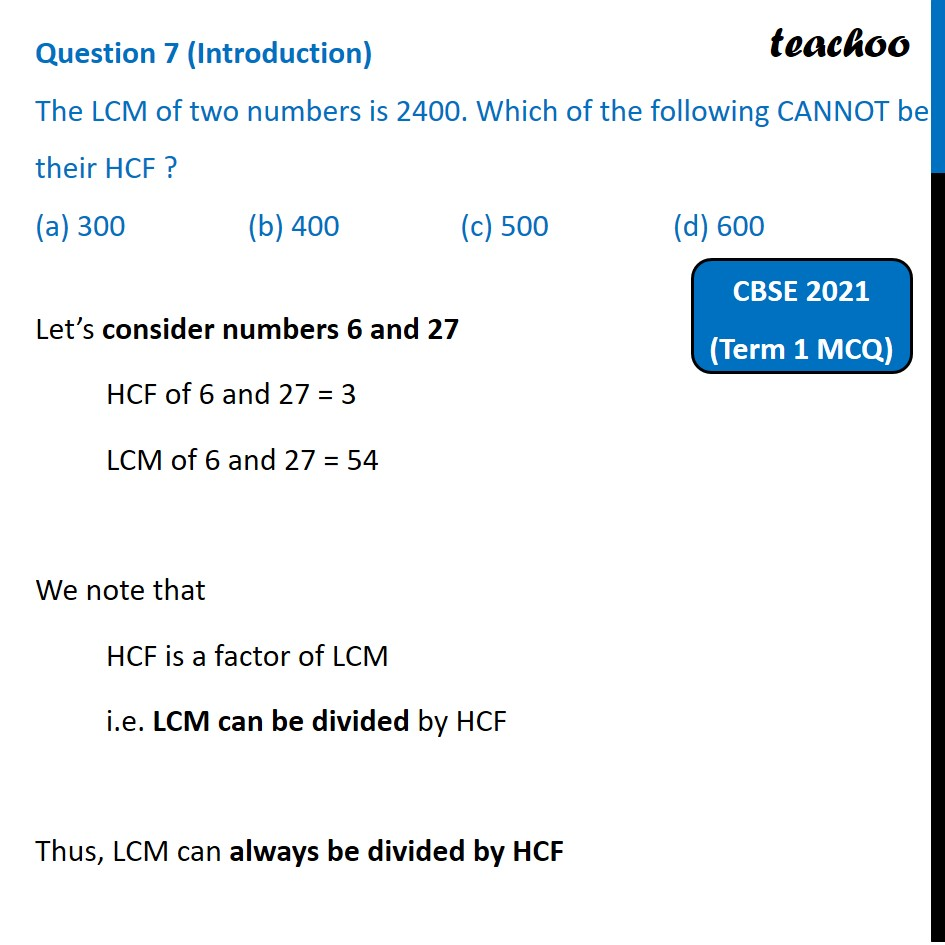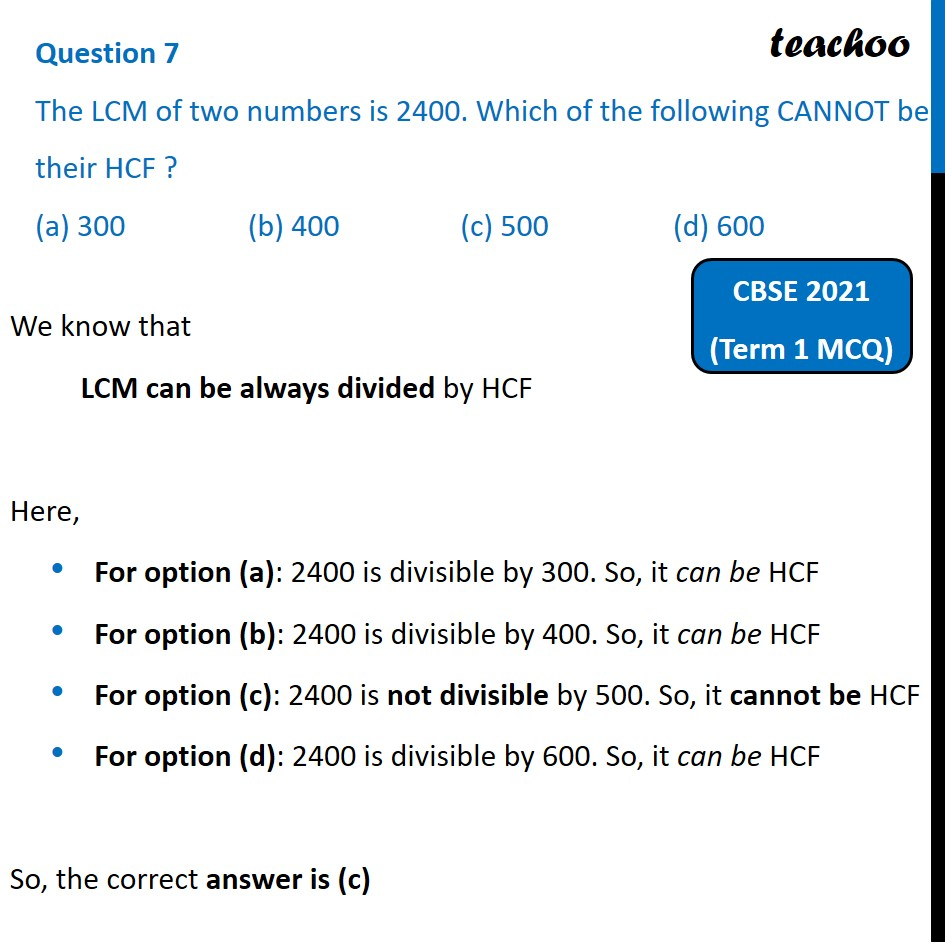Past Year MCQ (Maths Standard)

Chapter 1 Class 10 Real Numbers
Serial order wiseGet live Maths 1-on-1 Classs - Class 6 to 12

### Transcript

Question 7 (Introduction) The LCM of two numbers is 2400. Which of the following CANNOT be their HCF ? (a) 300 (b) 400 (c) 500 (d) 600Let’s consider numbers 6 and 27 HCF of 6 and 27 = 3 LCM of 6 and 27 = 54 We note that HCF is a factor of LCM i.e. LCM can be divided by HCF Thus, LCM can always be divided by HCF Question 7 The LCM of two numbers is 2400. Which of the following CANNOT be their HCF ? (a) 300 (b) 400 (c) 500 (d) 600We know that LCM can be always divided by HCF Here, For option (a): 2400 is divisible by 300. So, it can be HCF For option (b): 2400 is divisible by 400. So, it can be HCF For option (c): 2400 is not divisible by 500. So, it cannot be HCF For option (d): 2400 is divisible by 600. So, it can be HCF So, the correct answer is (c)